# 全息透镜与数字全息技术

## 传统全息术

### 基本原理

$$I(x,y)=|E_0|^2+2E_0\cos\big(\frac{\pi\rho^2}{\lambda z_0}\big)$$

$$\rho_m^2=m\lambda z_0 \quad m=1,2,3,\cdots$$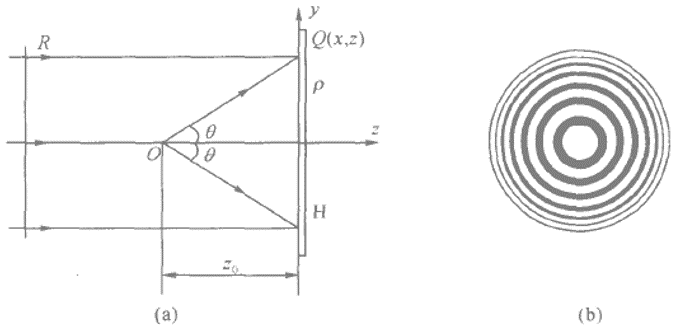◎ 图 1：同轴全息透镜的记录

$$\sin\theta'=\frac{\lambda}{\rho_1}$$

$$\frac{\lambda}{\rho_1}=\frac{\rho_1}{z_0}$$

$$\sin\theta'=\frac{\rho_1}{z_0}$$

$$\sin\theta\approx\tan\theta=\frac{\rho_1}{z_0}$$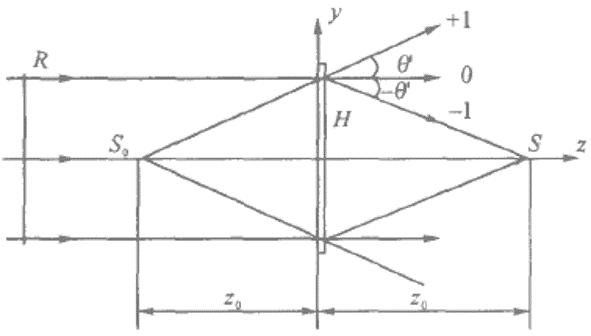◎ 图 2：全息波带片的衍射

1. 全息透镜具有与正透镜类似的聚焦特性。平行光透过全息透镜时能得到一个会聚球面波，会聚点即它的焦点。焦点至全息透镜的距离即为它的焦距。应当注意：全息透镜的焦距一般并不等于制作时形成球面波的透镜的焦距。它只取决于光束会聚点至全息干板的距离，在实验中可以调节这个距离。
2. 全息透镜也具有成像作用，其成像规律与普通透镜一致。

1. 由于正弦型薄全息图总是同时存在 ±1 级衍射，同一个全息透镜既相当于一个正透镜，同时也相当于一个负透镜。因此，同轴全息透镜在成像时，既有类似于正透镜所成的像，同时也有类似于负透镜所成的像。离轴全息透镜的成像是离轴的，可以把它看成是一个棱镜和一个透镜的组合。透镜起成像作用，棱镜则使光轴离轴偏转，表现出离轴全息透镜的成像和转像的双重功能，而普通透镜不能两者兼是。
2. 对于非线性记录的薄全息透镜，重现时除了 ±1 级衍射外，还同时有高次衍射，如 ±2 级、±3 级等衍射，因而全息透镜存在多重焦距和多重成像。
3. 全息透镜具有明显的色散作用。由于衍射角对应于不同波长的入射光有着不同的数值，所以同一个全息透镜即使是对于同一级衍射所形成的会聚焦点的位置也随波长的不同而变化。也就是说，对于不同的波长，全息透镜的焦距值也不同。表现出多焦点的特点。

### 搭建光路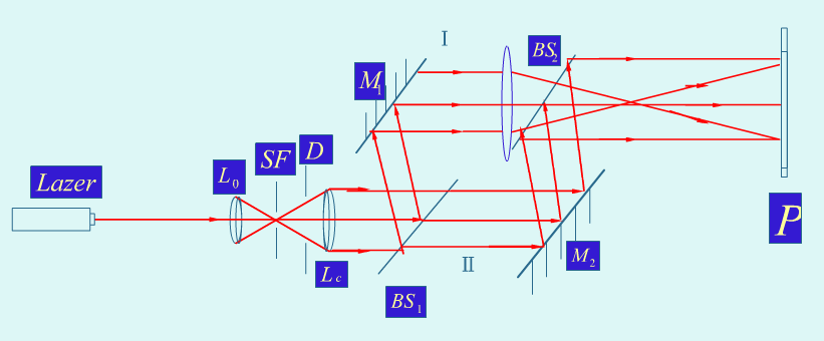◎ 图 3：马赫－泽德干涉仪

### 记录全息图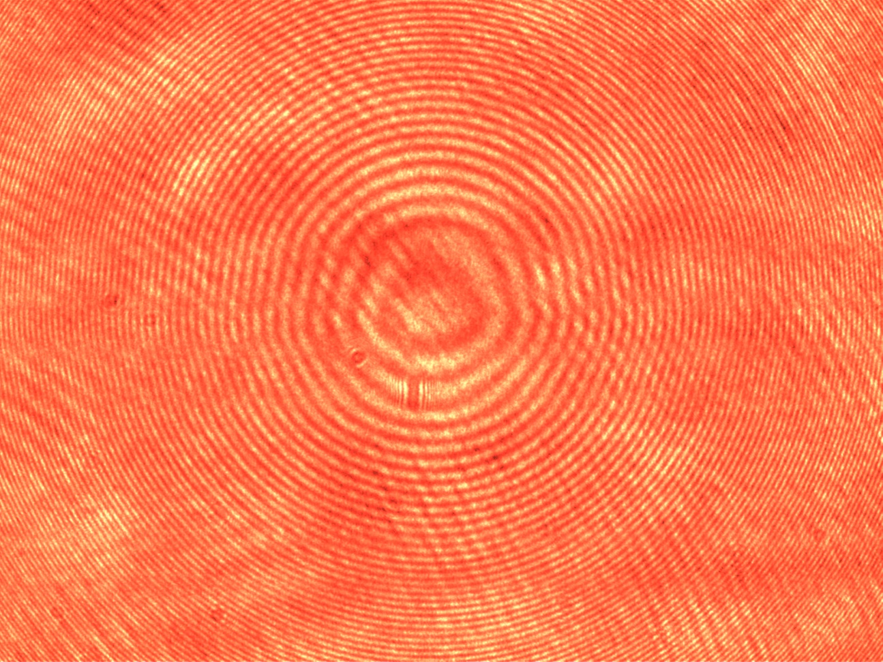◎ 图 4：采集到的全息图样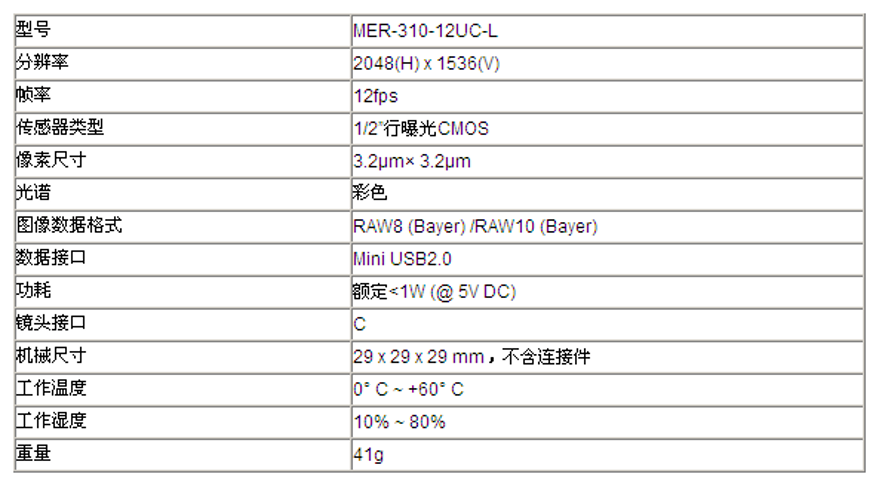◎ 图 5：工业相机参数

$$f=\frac{r_n^2}{2n\lambda}$$

200mm39.25cm19.25cm18.12cm

### 制作全息透镜

1. 显影：在 20℃±1℃ 的 D-19 显影液中漂洗 2 分钟左右，直到全息干板 $H$ 上出现灰色，在暗绿灯下观察到由灰变黑的现象，即可取出再用清水漂洗。线性记录振幅型全息图，最佳平均光密度为 0.6～1；
2. 停显：停显液中（20℃）停显 1 分钟左右，再用清水漂洗；
3. 定影：F-5 定影液（20℃）中定影 2 分钟左右，再水洗；
4. 漂白：在漂白液中漂白 1 分钟左右，可观察到全息干板上的黑色褪去，即可取出水洗。振幅型全息图经过氰化钾漂白剂得到相位型全息图，使衍射效率提高。应不停地漂动全息图，得呈乳白色薄层状平板。

## 数字全息术

### 基本原理

#### 数字全息图记录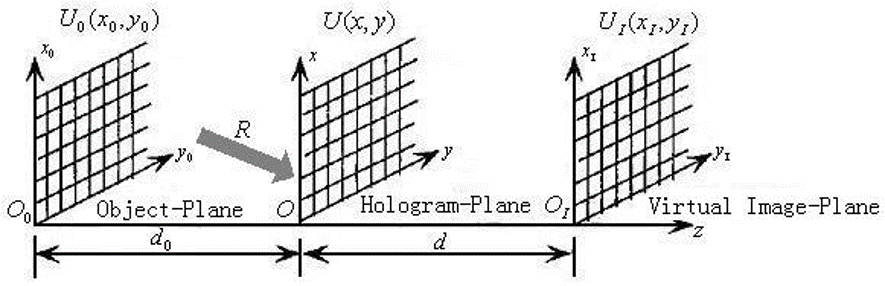◎ 图 6：数字全息图记录和再现的坐标系统变换示意图

$$O(x,y)=A_o(x,y)\exp[j\varphi_0(x,y)]$$

$$R(x,y)=A_r(x,y)\exp[j\varphi_r(x,y)]$$

\begin{aligned} I_H(x,y)=&|U(x,y)|^2=|O(x,y)+R(x,y)|^2\\ =&|A_o(x,y)|^2+|A_r(x,y)|^2+\\ &O(x,y)R^*(x,y)+O^*(x,y)R(x,y) \end{aligned}

$$I(u,v)=I_H(x,y)rect\big(\frac{x}{L_x},\frac{y}{L_y}\big)\sum_u\sum_v\delta(x-u\Delta x,y-v\Delta y)$$

$$\Delta e_{\min}=\frac{\lambda}{2\sin(\theta_{\max}/2)}$$

$$f_{\min}=\frac{2\sin(\theta_{\max}/2)}{\lambda}$$

$$\theta\leq\frac{\lambda}{2\Delta x}$$

$$\theta_{\max}=\frac{\lambda}{2\Delta x}$$

#### 数字全息图再现

\begin{aligned} U_I(x_I,y_I)=&\frac{\exp(jkd)}{j\lambda d}\iint_{-\infty}^{\infty}I(u,v)C(u,v)\\ &\exp\bigg\{\frac{jk}{2d}\big[(x_I-u)^2+(y_I-v)^2\big]\bigg\}dudv \end{aligned}

\begin{aligned} U_I(x_I,y_I)=&\frac{\exp(jkd)}{j\lambda d}\iint_{-\infty}^{\infty}I(u,v)C(u,v)\exp\bigg[\frac{jk}{2d}(u^2+v^2)\bigg]\\ &\exp\bigg[-j2\pi\frac{1}{\lambda d}(ux_1+vy_1)\bigg]dudv \end{aligned}

\begin{aligned} U_I(x_I,y_I)=&\frac{\exp(jkd)}{j\lambda d}\exp\bigg[-2\frac{j\pi}{\lambda d}(x_1^2+y_1^2)\bigg]\times\\ &F^{-1}\bigg\{I(u,v)C(u,v)\exp\bigg[-\frac{jk}{\lambda d}(u^2)+v^2\bigg]\bigg\} \end{aligned}

\begin{aligned} U_I(x_I,y_I)=&\frac{\exp(jkd)}{j\lambda d}\exp\bigg[\frac{j\pi}{\lambda d}(x_1^2+y_1^2)\bigg]\times\\ &F^{-1}\bigg\{I(u,v)C(u,v)\exp\bigg[\frac{jk}{\lambda d}(u^2)+v^2\bigg]\bigg\} \end{aligned}

\begin{aligned} I_I(x_I,y_I)&=U_I(x_I,y_I)U_I^*(x_I,y_I)\\ \Phi_I(x_I,y_I)&=\arctan\frac{Im[U_I(x_I,y_I)]}{Re[U_I(x_I,y_I)]} \end{aligned}

### 计算模拟全息记录与再现

#### 物面与全息图抽样

$$f(x,y)=a(x,y)\exp[i\varphi(x,y)]$$

$$F(u,v)=A(u,v)\exp[i\varphi(u,v)]$$

\begin{aligned} f(x,y)=0\quad|x|\geq\frac{\Delta x}{2},|y|\geq\frac{\Delta y}{2}\\ F(u,v)=0\quad|u|\geq\frac{\Delta u}{2},|v|\geq\frac{\Delta v}{2} \end{aligned}

\begin{aligned} \delta x\geq\frac{1}{\Delta u},\quad\delta y\geq\frac{1}{\Delta v}\\ \delta u\geq\frac{1}{\Delta x},\quad\delta v\geq\frac{1}{\Delta y} \end{aligned}

$$f(m,n)\stackrel{FFT}{\longrightarrow}F(j,k)=F_r(j,k)=iF_i(j,k)$$

\begin{aligned} A(j,k)=\sqrt{F_r^2(j,k)+F_i^2(j,k)}\\ \varphi(j,k)=\arctan\bigg[\frac{F_i(j,k)}{F_r(j,k)}\bigg] \end{aligned}

### 空间光调制器实时再现

#### 空间光调制器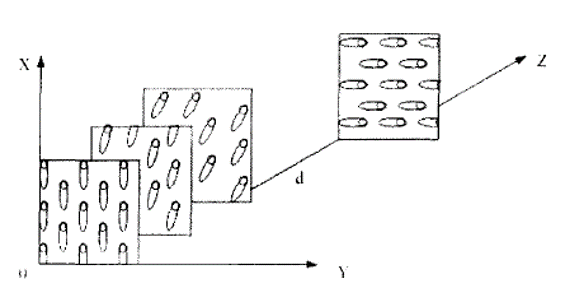◎ 图 7：TNLC 分层模型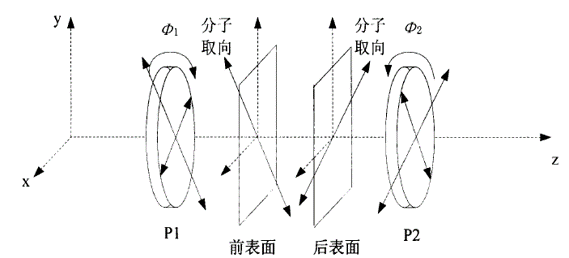◎ 图 8：SLM 光路示意图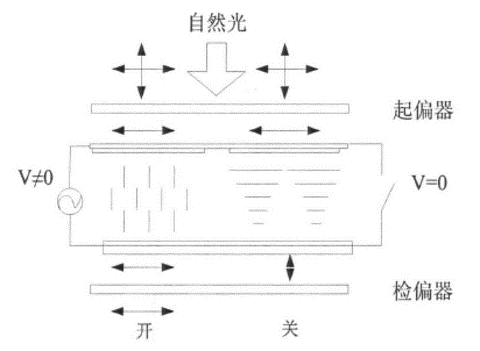◎ 图 9：液晶屏的透光原理

### 实验记录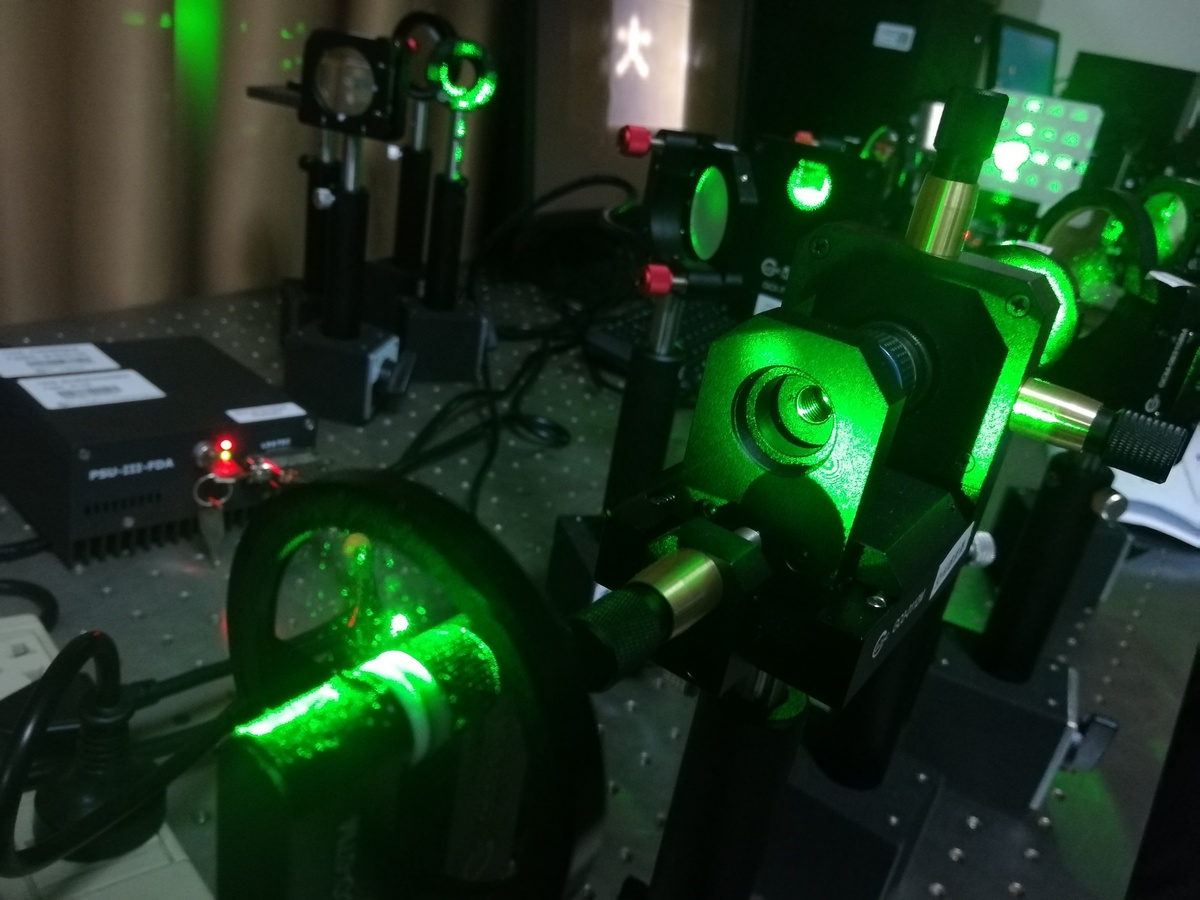◎ 图 10：实验中搭建的全息光路

#### 计算机模拟全息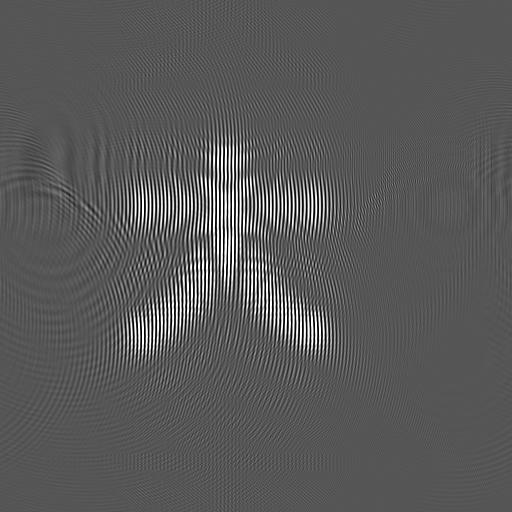◎ 图 11：数字记录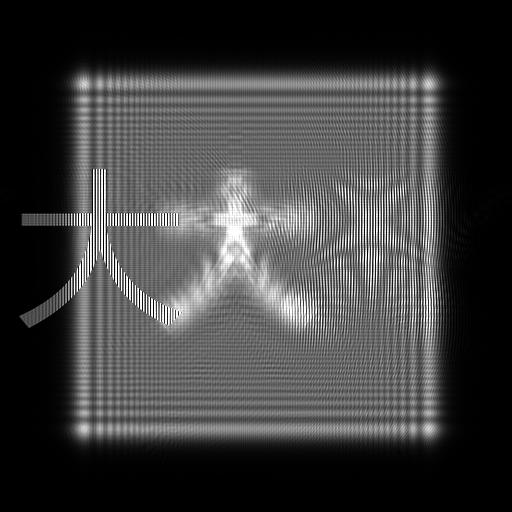◎ 图 12：数字再现

#### 数字全息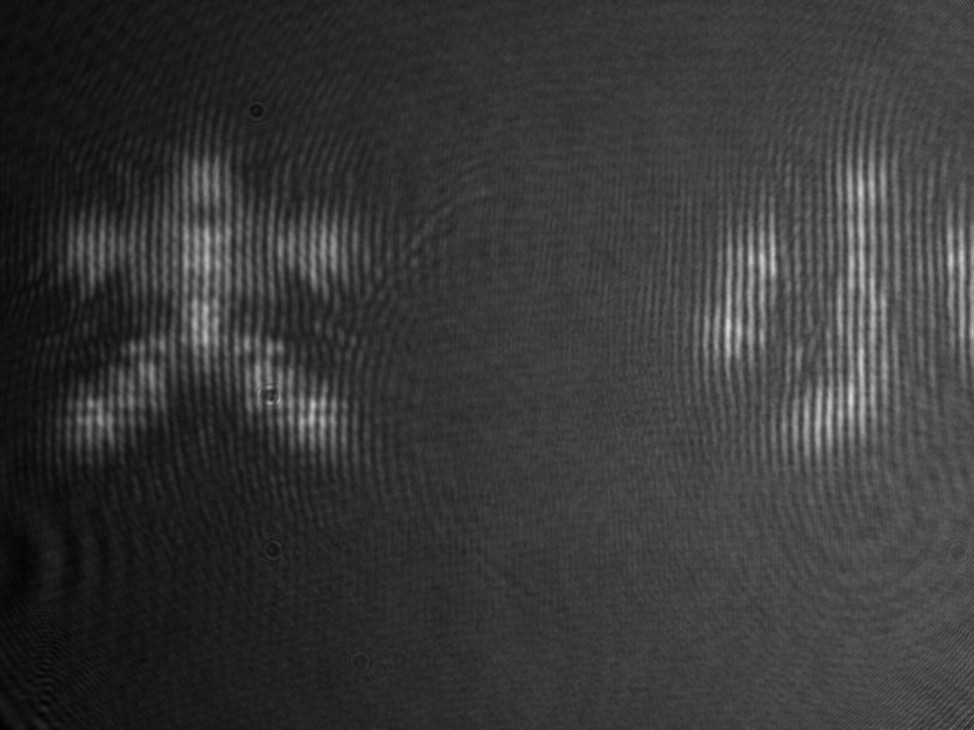◎ 图 13：光学记录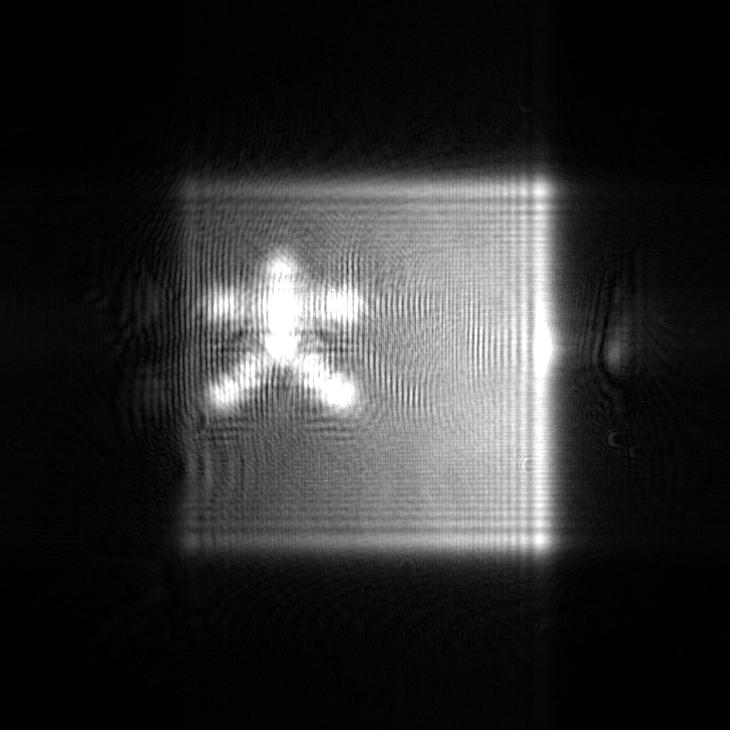◎ 图 14：数字再现

#### 可视数字全息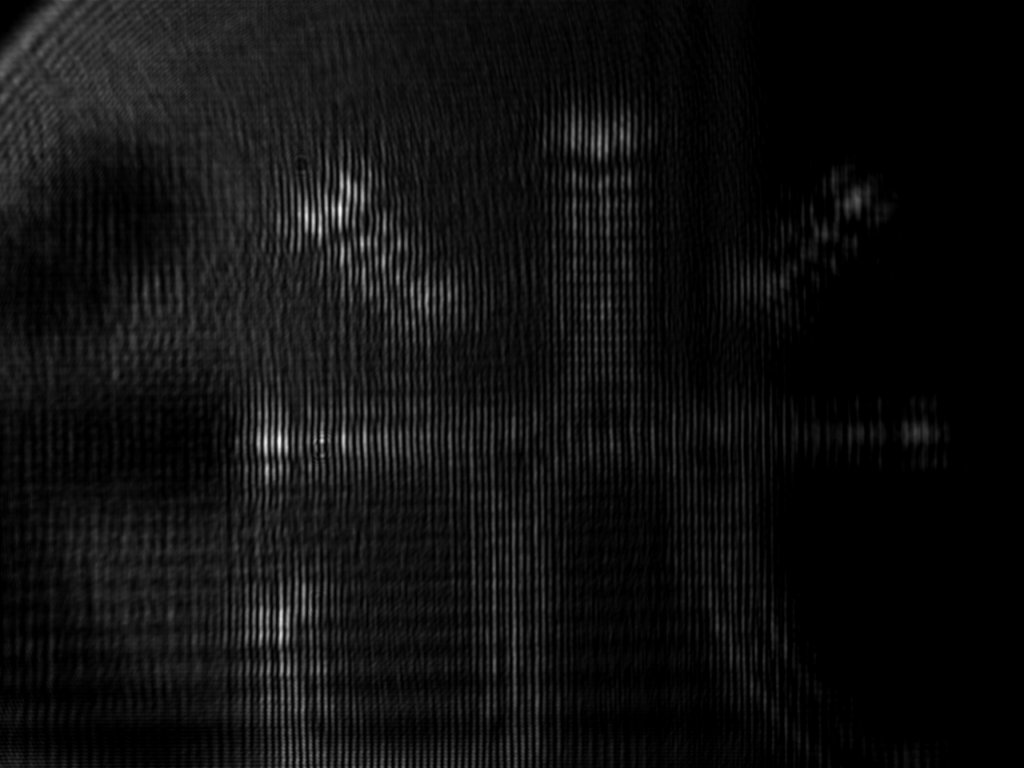◎ 图 15：光学再现

#### 实时传统全息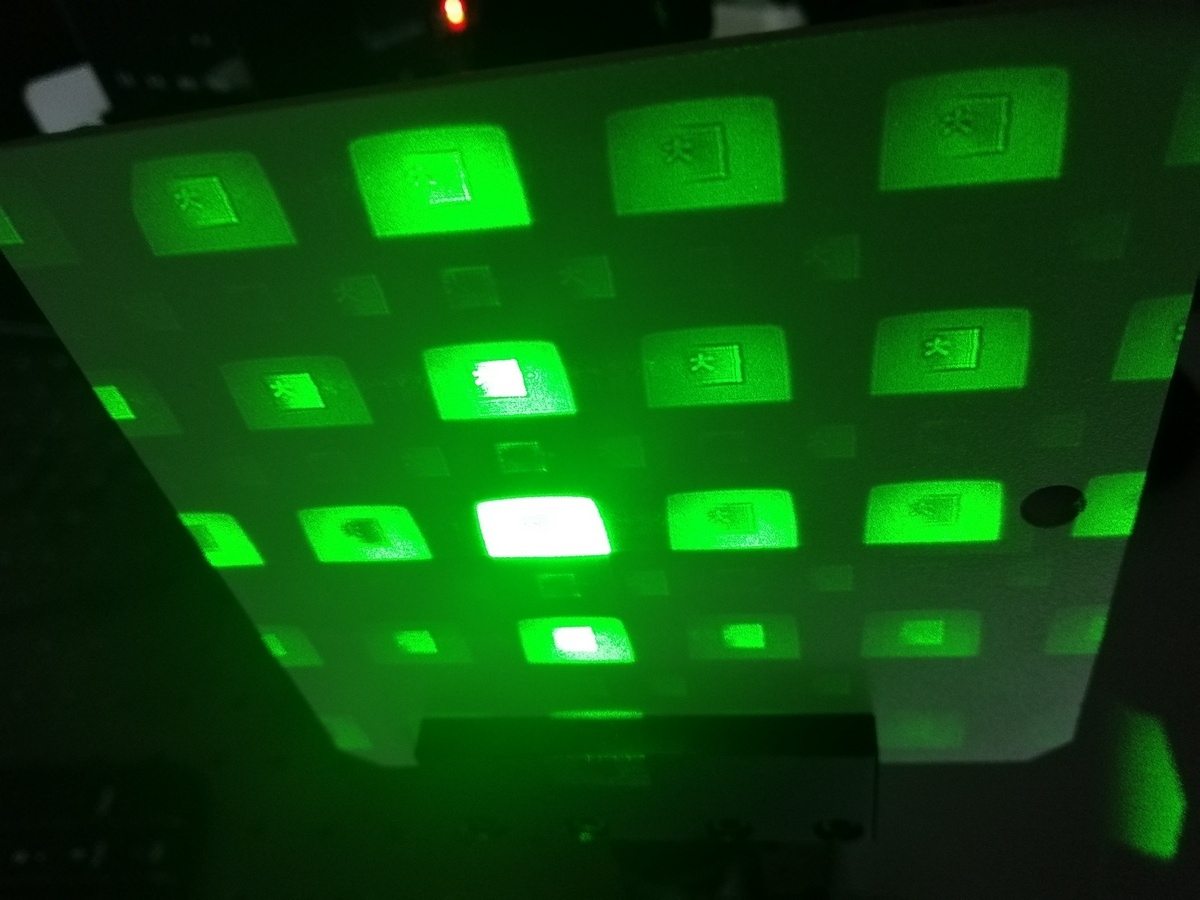◎ 图 16：光学再现